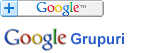### Căutare în Webster - Dicționarul explicativ al limbii engleze

 Cuvânt
Pentru căutare rapidă introduceți minim 3 litere.

## AXIS - Definiția din dicționar

Notă: Puteţi căuta fiecare cuvânt din cadrul definiţiei printr-un simplu click pe cuvântul dorit.

Ax"is (&unr_;), n. [L.] (Zo&ö;l.) The spotted deer (Cervus axis or Axis maculata) of India, where it is called and parrah (Moorish name).
[1913 Webster]

Ax"is (&unr_;), n.; pl. Axes (&unr_;). [L. axis axis, axle. See Axle.]
[1913 Webster]

2. (Math.)
[1913 Webster]

3. (Bot.) Gray.
[1913 Webster]

4. (Anat.) (a) The second vertebra of the neck, or vertebra dentata. (b)
[1913 Webster]

5. (Crystallog.)
[1913 Webster]

6. (Fine Arts)
[1913 Webster]

Anticlinal axis (Geol.), a line or ridge from which the strata slope downward on the two opposite sides. -- Synclinal axis, a line from which the strata slope upward in opposite directions, so as to form a valley. -- Axis cylinder (Anat.), the neuraxis or essential, central substance of a nerve fiber; -- called also , , and . -- Axis in peritrochio, the wheel and axle, one of the mechanical powers. -- Axis of a curve (Geom.), a straight line which bisects a system of parallel chords of a curve; called a principal axis, when cutting them at right angles, in which case it divides the curve into two symmetrical portions, as in the parabola, which has one such axis, the ellipse, which has two, or the circle, which has an infinite number. The two axes of the ellipse are the major axis and the minor axis, and the two axes of the hyperbola are the transverse axis and the conjugate axis. -- Axis of a lens, the straight line passing through its center and perpendicular to its surfaces. -- Axis of a microscope or Axis of a telescope, the straight line with which coincide the axes of the several lenses which compose it. -- Axes of co&ö;rdinates in a plane, two straight lines intersecting each other, to which points are referred for the purpose of determining their relative position: they are either rectangular or oblique. -- Axes of co&ö;rdinates in space, the three straight lines in which the co&ö;rdinate planes intersect each other. -- Axis of a balance, that line about which it turns. -- Axis of oscillation, of a pendulum, a right line passing through the center about which it vibrates, and perpendicular to the plane of vibration. -- Axis of polarization, the central line around which the prismatic rings or curves are arranged. Brewster. -- Axis of revolution (Descriptive Geom.), a straight line about which some line or plane is revolved, so that the several points of the line or plane shall describe circles with their centers in the fixed line, and their planes perpendicular to it, the line describing a surface of revolution, and the plane a solid of revolution. -- Axis of symmetry (Geom.), any line in a plane figure which divides the figure into two such parts that one part, when folded over along the axis, shall coincide with the other part. -- Axis of the equator, ecliptic, horizon (or other circle considered with reference to the sphere on which it lies), the diameter of the sphere which is perpendicular to the plane of the circle. Hutton. -- Axis of the Ionic capital (Arch.), a line passing perpendicularly through the middle of the eye of the volute. -- Neutral axis (Mech.), the line of demarcation between the horizontal elastic forces of tension and compression, exerted by the fibers in any cross section of a girder. -- Optic axis of a crystal, the direction in which a ray of transmitted light suffers no double refraction. All crystals, not of the isometric system, are either uniaxial or biaxial. -- Optic axis, Visual axis (Opt.), the straight line passing through the center of the pupil, and perpendicular to the surface of the eye. -- Radical axis of two circles (Geom.), the straight line perpendicular to the line joining their centers and such that the tangents from any point of it to the two circles shall be equal to each other. -- Spiral axis (Arch.), the axis of a twisted column drawn spirally in order to trace the circumvolutions without. -- Axis of abscissas and Axis of ordinates. See Abscissa.
[1913 Webster]